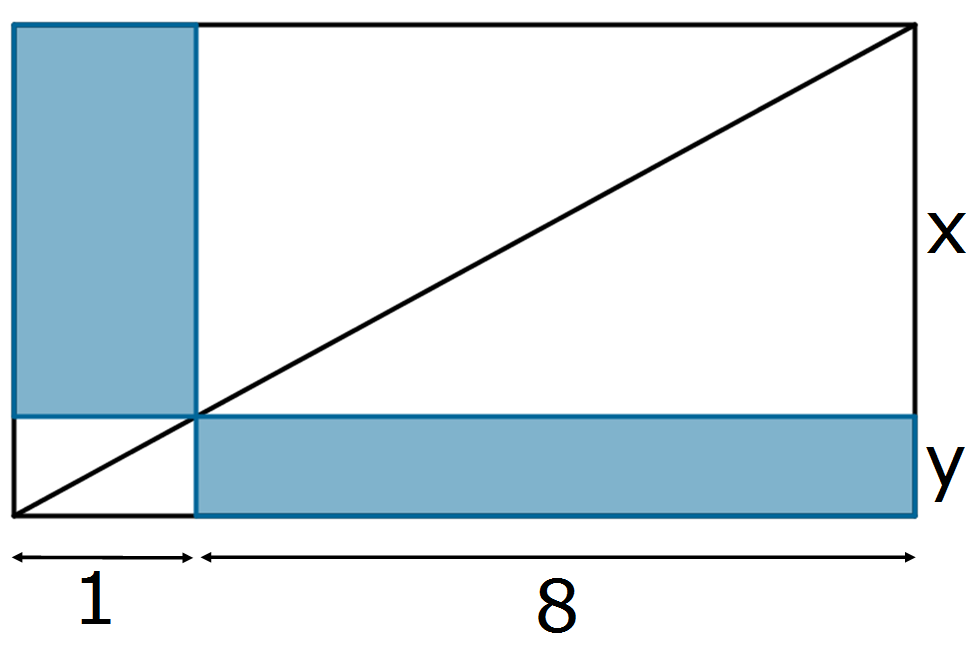#### You may also like### Rationals Between...

What fractions can you find between the square roots of 65 and 67?### There's a Limit

Explore the continued fraction: 2+3/(2+3/(2+3/2+...)) What do you notice when successive terms are taken? What happens to the terms if the fraction goes on indefinitely?### Not Continued Fractions

Which rational numbers cannot be written in the form x + 1/(y + 1/z) where x, y and z are integers?

# Diagonal Touch

##### Age 14 to 16 Short Challenge Level:$\frac{16}{81}$ is shaded.

Let $x$ and $y$ be the distances shown. Then the shaded area is $8y + x$. But there are a number of similar triangles and from one pair $${x\over8} = {y\over1}$$ i.e. $$x = 8y$$ So, $$\frac{\mbox{shaded area}}{\mbox{total area}} = \frac{8y + x}{9(x + y)} = \frac{8y + 8y}{9 \times 9y} = \frac{16}{81}$$
This problem is taken from the UKMT Mathematical Challenges.
You can find more short problems, arranged by curriculum topic, in our short problems collection.